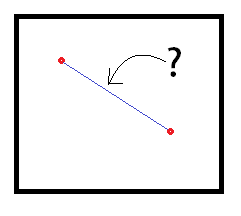# Average Distance Between Two PointsSuppose you have a $1\times 1$ square. If two points are randomly picked within the square, what is the expected value (average) of the distance between them, rounded to 4 decimal places?

×